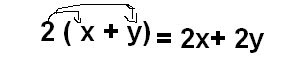## Thursday, September 4, 2008

### PolynomialsToday in class we started with polynomials which is our first Unit of the course.

Mr. Kurapatwa thought as a little bit of Definition of Polynomials.

As we know that our first unit is polynomial, So we have to know the definition of polynomial!

1. Polynomials: is an algebraic expression of one or more terms
e.g. 2x+3y or x^2 - 5x+4

2. Monomial: is an algebraic expression of one term
e.g. 6x or 5ab or y

3. Binomial: is an algebraic expression of two terms
*We have to remember that any expression with two terms is a Binomial
e.g. x-y or x + 4

4. Trinomial: is an algebraic expression of three terms
e.g. 2x + 5y -4

*We also learn 5 other Definition*

1) Terms: is a symbol or group of symbols separated from other symbols bya plus or minus sign.
e.g. 3x - 5y + 7xyz in this expression we know that there are three terms, note that terms may contain several numbers and letters.

2) Coefficient: The numeric factor of a term (a number with a variable)
* for example 3x + 5y -4* the coefficient in this expression are 5 & 4

3) Sum: The result of Adding two or more number
e.g. The sum of 2 and 3 is? so we know that if we add 2 and 3 we get 5

4) Product: The result of Multiplying two or more numbers
e.g. the product of 7 and 3 is? so what we will do is to multiply 7 and 3, which will give us 49.

Time to Practice!!

a. 7x-y-2x+3y? (when we are adding or subtracting polynomials we combine the like terms which looks the same.)
7x-2x are like terms
3y-y are like terms
so now it's easy for as to solve it!

2. (4x-2y-4)-(-3x-2y+5) *i think this is little bit trickier*
What we will do hear is multiply the minus to each of the second expression on the right hand.
And now we remain with (4x-2y-4)+(3x+2y-5)
now the second expression is changed, what we will do is to take off the brackets and as i say we combine the like terms.
4x+3x-2y+2y-4-5
7x-9 ans

Multiplying and Dividing of Polynomials.

1. 2(x+y)we just multiplied 2 to everything in the brackets
2.Now what we have to is to divide
the coefficient and cancel the like terms
variable. Which Will give as 8x

For More Practice and Exercise: